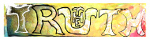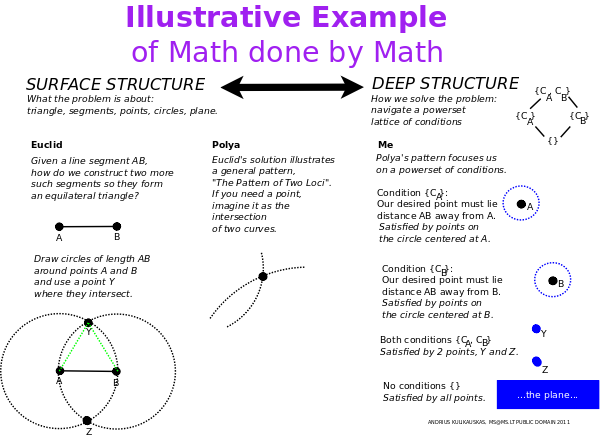发现 ms@ms.lt +370 607 27 665 My work is in the Public Domain for all to share freely. Software Discovery in Mathematics: A System of Deep Structure I will talk about how we may systematically study the ways of figuring things out in mathematics.George Polya, in his book, "How to Solve It", considers Euclid's problem of how to construct an equilateral triangle. If we are given the side AB, how do we construct the other two? The solution is a recurring idea which Polya calls the "pattern of two loci". We think of there being two separate conditions. One side must extend a length AB from the point A. Another side must extend a length AB from the point B. We thus draw two circles of radius AB centered at A and B. The points where the two circles intersect are those where we can draw a third point C which satisfies both conditions so that our triangle is equilateral. I realized that our minds solve this problem by imagining a powerset lattice of conditions. Circle A is one condition, circle B is another condition, and the intersection of A and B satisifies the union of these two conditions. Our minds have thus solved the surface problem (constructing a triangle) by considering a simpler, deeper structure (a lattice of conditions). This brings to mind linguist Noah Chomsky's work in syntax and architect Christopher Alexander's work on pattern languages. I collected such problem solving patterns discussed in Paul Zeitz's book "The Art and Craft of Problem Solving" and other sources. Each distinct pattern makes use of a structure which is familiar to mathematicians and yet is not explicit but mental. We may consider those math structures to be cognitively "natural" which are used by the mind in solving math problems. I present to you 24 patterns which I identified and systematized them in a way which suggests they are complete. Let me first show how to systematically generate the 4 infinite families of polytopes, An (simplexes), Cn (cross polytopes), Bn (hypercubes), Dn (demicubes), whose symmetry groups are also the Weyl groups for the root systems of the classical Lie algebras. The resulting structures, actions and relationships will help me talk systematically about the mathematical ways of figuring things out. Imagine the Center of a polytope. The simplexes An are generated by adding one vertex at a time, along with edges to all of the existing vertices, which makes clear that it is indeed a new vertex. The Center has no vertices and its dual is the Totality of all vertices. The cross polytopes Cn are generated when the Center adds two vertices at a time, not connected to each other, yet connected to all other vertices. Cross polytopes have a Center but no Totality. The cubes Bn have a Totality but no Center. They are generated from the Totality as an unfolding sequence of perpendicular mirrors. The demicubes Dn have no Center and no Totality. They are coordinate systems which are like a cube that has been folded in half, and also like a simplex but with double edges and a distinguished vertex, the Origin. The polytopes An, Cn, Bn and Dn are the foundations for 4 different geometries and metalogics. The simplexes An span all of space from the Center and so are the basis for affine geometry where directions are preserved. They model whether things are true as in proof by contradiction. The cross-polytopes Cn define opposite directions and so are the basis for projective geometry where lines are preserved. They let us model what is true by singling out the most relevant dimensions as with "wishful thinking". The cubes Bn relate perpendicular directions and so are the basis for conformal geometry where angles are preserved. They let us figure out how things are true by working backwards from a multidimensional point to the spaces which are its conditions. The coordinate systems Dn superimpose directions and angles and so are the basis for symplectic geometry where areas are preserved. They let us figure out why things are true by interpreting variables in ways that clarify the semiotic disconnects between these four geometries. For example, drawing a picture can help us interpret variables as dependent vs. independent, known vs. unknown, given vs. arbitrary, fixed vs. varying, concrete vs. abstract, defined vs. undefined and so on. We can think of each of these four geometries as relating the explicit math on a sheet of paper and the implicit math by which we interpret it with our minds. One way to figure things out is to realize that we can always start with a fresh sheet. A second way is to completely structure our sheet so that our mind can act on it like a symmetry group, constructing strings of actions and undoing them at will. Finally, at third ways is to realize that our knowledge is limited by context. If I ask you, what is 10+4? You may say 14, but the answer is 2, because I am thinking about a clock. Even so, we seek a symmetric group which brings together algebraic structures and analytic dynamics. The four polytopes An, Cn, Bn, Dn illustrate how algebraic structure clarifies problems. The simplex suggests that we can solve a problem by imagining the center of the whole. The cross-polytopes suggest that we make use of parity, for example, to compare what is in a set and what is in its complement. The cubes suggest that we can compare sums of amounts and units. The coordinate systems suggest that we can construct or deconstruct a vector subspace in an orderly manner given a list of canonical basis elements. The four polytopes also inspire dynamic analytic perspectives, though not within a single sheet, but through a sequence of sheets. We can apply mathematical induction, as suggested by the center of a simplex, in that it ever generates the next vertex. We can imagine a maximum and minimum in any dimension, as suggested by the cross-polytopes. The quadrants of the cubes help us imagine that various solutions can serve as least upper bounds or greatest lower bounds. And the twofold nature of the coordinate systems Dn let us imagine that if we generate a sequence of vertices, then it will ultimately achieve its limit somewhere in the half cube. We also imagine functors which couple the algebraic structural and analytical dynamic points of view through the scientific method of taking a stand, following through and reflecting. Given an addition formula (2**X)(2**Y) = 2**(X+Y), we take a stand by extending the domain so that the formula makes sense for zero, negative integers, fractions and so on. Next, we vary these variables continuously to learn whether our model breaks or holds. Finally, we can generalize by considering our constraint as a recurrence relation and superimposing it upon itself, as with a generating function. Once we have our symmetry group and a comprehensive system, then our four geometries let us imagine metalogics which identify perspectives outside and inside the mathematical system. But symmetry also lets us establish looser equivalences through six logical transformations which apply a system's analytic dynamics to a subsystem's algebraic structure. We can illustrate these transformations with different ways of interpreting multiplication. Our mind visualizes fractal self-similarities in recopying a whole, as multiplying powers of two. We link global and local proportions in rescaling the whole. We rescale multiples by tallying and skip counting and thereby leverage the total order. We redistribute a set with box multiplication to disentangle "ands" and "ors" and organize a power set lattice of conditions. We redistribute multiples by factoring components, regrouping and recounting ordinals. We redistribute a whole with long division, where we keep having a remainder, creating a walk without cycles. In summary, the mathematical ways of figuring things aspire to construct a symmetry group by which we can play with a system. The symmetry group and its system are established by stitching together structural algebraic and dynamical analytic perspectives through a cycle of extending a domain, varying continuously and selfsuperimposing a recurrence relation. The system is defined with respect to four geometries which serve as metalogics to identify perspectives outside and inside the system. The symmetry group relates the different geometries with transformations which link a system's perspective with a restructured subsystem's perspective. These transformations define a loose equivalence which allows us to reinterpret all context and ever start fresh with a blank sheet. Mathematics is thus systematic but inherently flexible. Naujausi pakeitimai Introduction E9F5FC Understandable FFFFFF Questions FFFFC0 Notes EEEEEE 维基百科 影片 书 读物
 Puslapis paskutinį kartą pakeistas 2016 birželio 20 d., 12:09